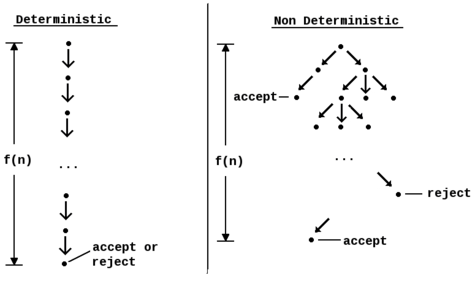# Nondeterministic algorithmA deterministic algorithm that performs f(n) steps always finishes in n steps and always returns the same result. A non deterministic algorithm that has f(n) levels might not return the same result on different runs. A non deterministic algorithm may never finish due to the potentially infinite size of the fixed height tree.

In computer science, a nondeterministic algorithm is an algorithm that, even for the same input, can exhibit different behaviors on different runs, as opposed to a deterministic algorithm. There are several ways an algorithm may behave differently from run to run. A concurrent algorithm can perform differently on different runs due to a race condition. A probabilistic algorithm's behaviors depends on a random number generator. An algorithm that solves a problem in nondeterministic polynomial time can run in polynomial time or exponential time depending on the choices it makes during execution. The nondeterministic algorithms are often used to find an approximation to a solution, when the exact solution would be too costly to obtain using a deterministic one.

The notion was introduced by Robert W. Floyd.

## Use

Often in computational theory, the term "algorithm" refers to a deterministic algorithm. A nondeterministic algorithm is different from its more familiar deterministic counterpart in its ability to arrive at outcomes using various routes. If a deterministic algorithm represents a single path from an input to an outcome, a nondeterministic algorithm represents a single path stemming into many paths, some of which may arrive at the same output and some of which may arrive at unique outputs. This property is captured mathematically in "nondeterministic" models of computation such as the nondeterministic finite automaton. In some scenarios, all possible paths are allowed to run simultaneously.

In algorithm design, nondeterministic algorithms are often used when the problem solved by the algorithm inherently allows multiple outcomes (or when there is a single outcome with multiple paths by which the outcome may be discovered, each equally preferable). Crucially, every outcome the nondeterministic algorithm produces is valid, regardless of which choices the algorithm makes while running.

In computational complexity theory, nondeterministic algorithms are ones that, at every possible step, can allow for multiple continuations (imagine a man walking down a path in a forest and, every time he steps further, he must pick which fork in the road he wishes to take). These algorithms do not arrive at a solution for every possible computational path; however, they are guaranteed to arrive at a correct solution for some path (i.e., the man walking through the forest may only find his cabin if he picks some combination of "correct" paths). The choices can be interpreted as guesses in a search process.

A large number of problems can be conceptualized through nondeterministic algorithms, including the most famous unresolved question in computing theory, P vs NP.

## Implementing nondeterministic algorithms with deterministic ones

One way to simulate a nondeterministic algorithm N using a deterministic algorithm D is to treat sets of states of N as states of D. This means that D simultaneously traces all the possible execution paths of N (see powerset construction for this technique in use for finite automata).

Another is randomization, which consists of letting all choices be determined by a random number generator. The result is called a probabilistic deterministic algorithm.

## Examples

### Primality testing

The problem: given a natural number n larger than two, determine whether it is prime.

A nondeterministic algorithm for this problem is the following based on Fermat's little theorem:

1. Repeat thirty times:
1. Pick a random integer a such that 2 ≤ an-1.
2. If, return answer composite